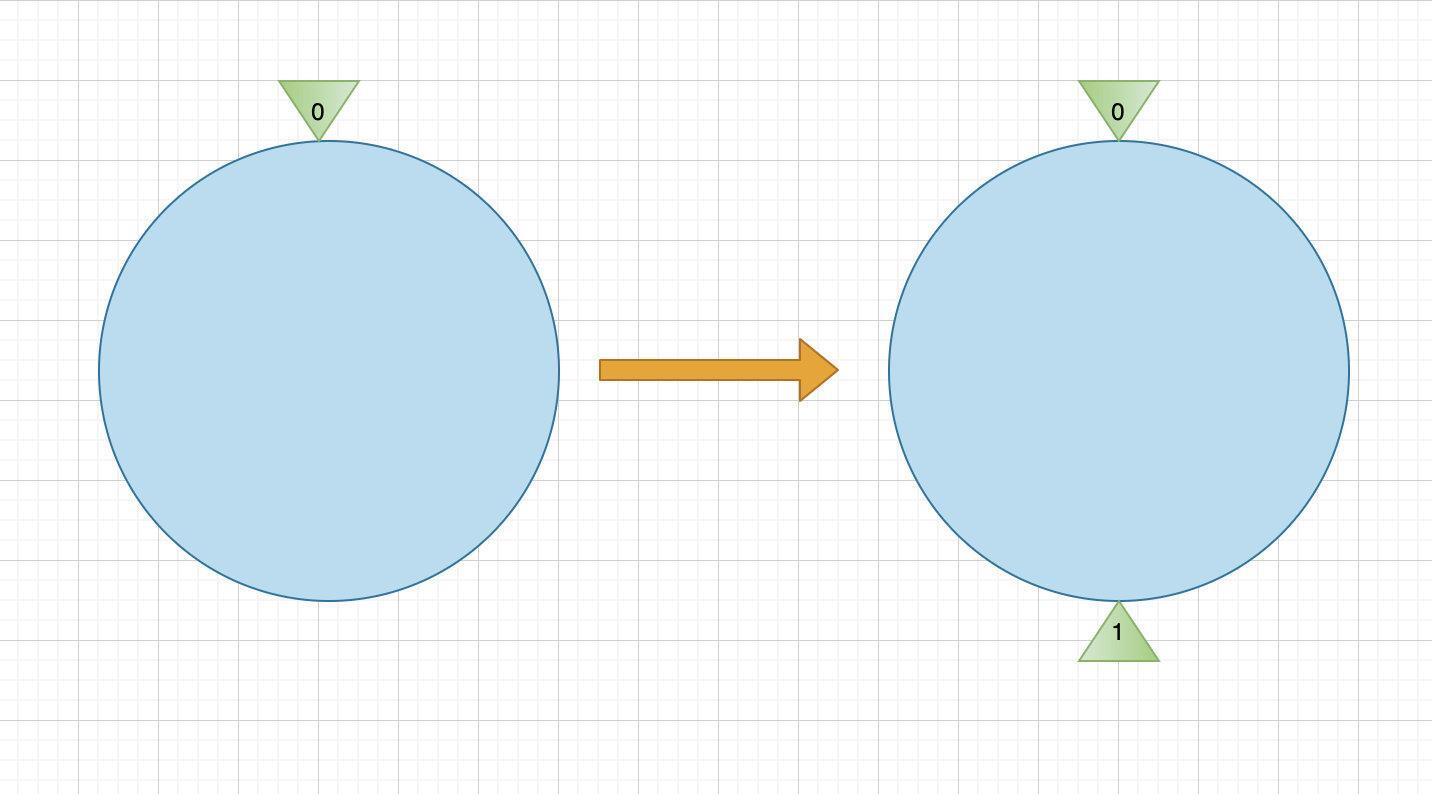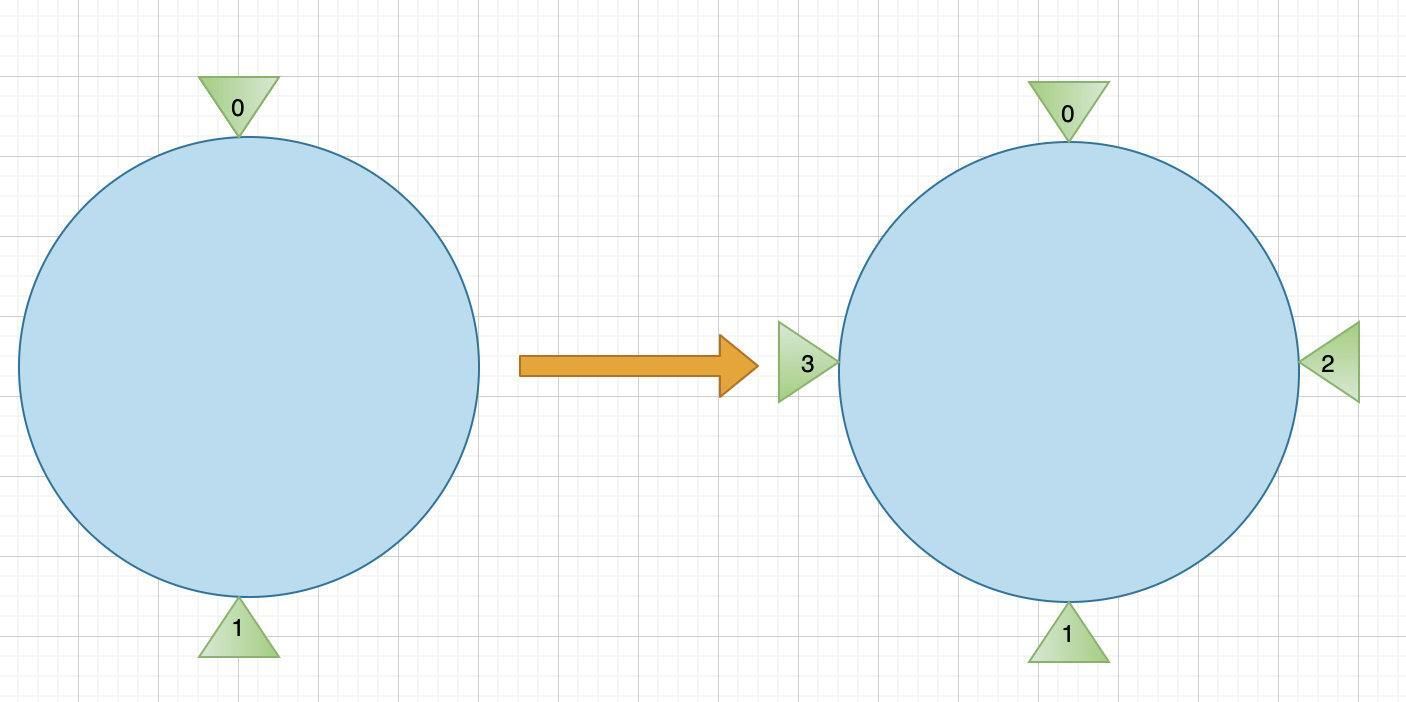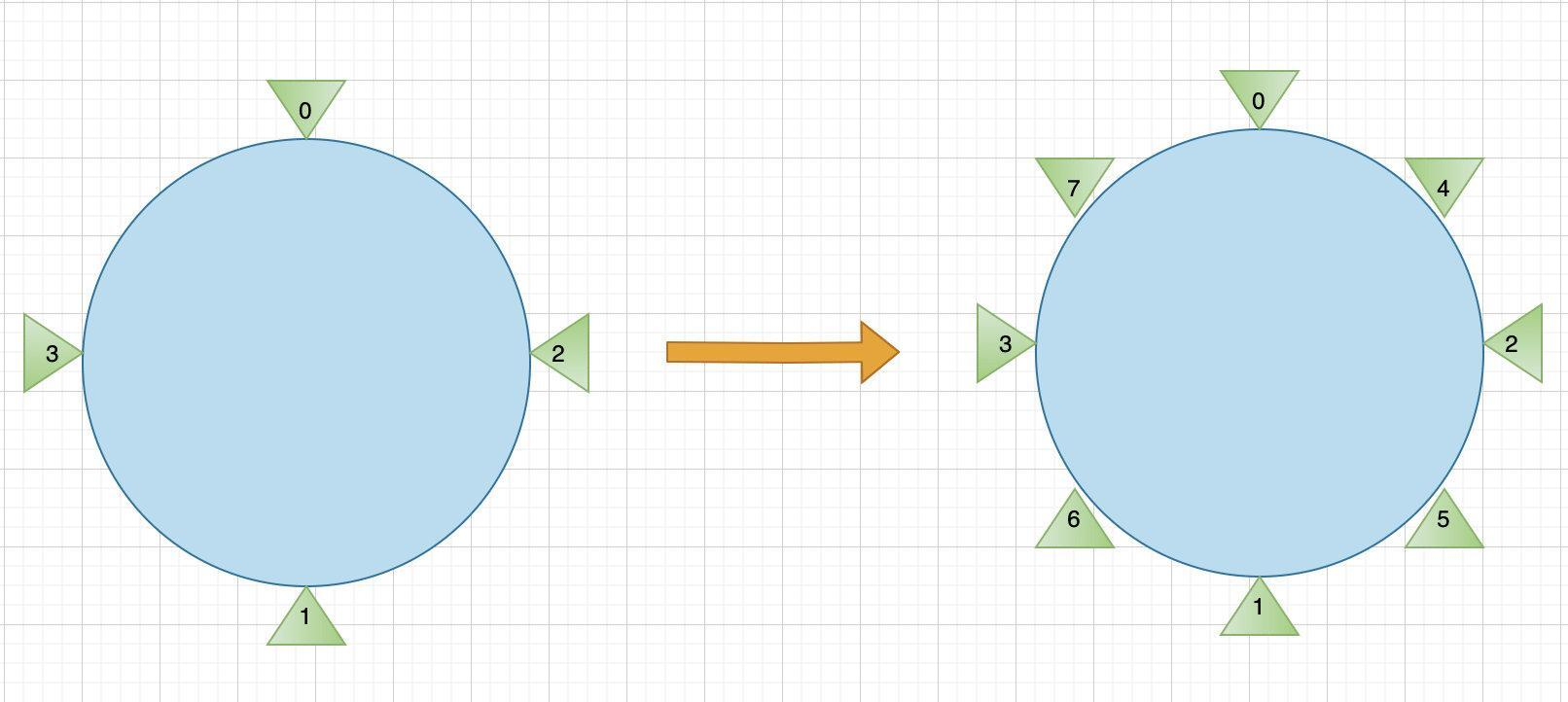# 2N 方定点算法### ﻿

2N方定点算法，是一次机缘巧合 针对DB层水平扩容的定制的算法。支持流量均衡，扩容节点流量只需偏移一台DB机器。

﻿

### 缘起

﻿

﻿

• DB层采用Redis做主库，MySQL做Redis异构库(用于数据持久化)

﻿

• 比赛系统，写多读少，而且流量峰值到来可预估

﻿

• 用户平时不会使用系统，只有比赛期间才使用

﻿

### 分析

﻿

﻿

﻿

﻿

(1)比对哈希环的代码有个bug ﻿(2)虚拟节点设置的量不够

﻿

• 一致性哈希算法的节点使用ip来计算，而我们采用序号。

﻿

﻿

• 一致性哈希算法的流量，是基于用户ip或者服务器的IP来计算哈希环，而我们使用用户ID

﻿

﻿

﻿

﻿

﻿



1. 同个用户的请求没有落在不同节点导致的读写一致性问题

2. 某个节点如果挂了，只影响这个节点的用户。其他节点的用户不受影响。



﻿

﻿

> 对一段连续的ID，如何均衡到各个节点成了这个问题的关键

﻿

### 实现

﻿

﻿

﻿

﻿

#### 节点变化图：

﻿

• 1个节点扩展为两个

﻿﻿

• 两个节点扩充为4个﻿

• 4个节点扩容为8个

﻿﻿

﻿

﻿

#### 节点位置

﻿

﻿

1. 两个节点的时候，节点分布在环的 原点和1/2 处。2. 第三个节点的来的时候，插入在1/4处，第四个节点插入在3/4处3. 第五个节点在1/8 ....4. 1/2 = 2/4 = 4/8 也就是节点按2分法插入，插满对应位置。   再来节点，再做一次2等分切分，原先的节点位置不变，但是新切分位置，多处于本轮新增切分点的1/2处

﻿

﻿

f(k) = (2(k-(2^level-1) +1)/2^level)*2^n      = (2k-(2^level) +1) * (2^n/2^level)      = (2k-(2^level) +1) * (2 ^ (n-level))h(k)  = level

﻿

﻿

﻿

#### 用户ID离散

﻿

﻿

﻿

﻿

﻿

﻿

﻿

﻿

﻿

L(uid) = uid % 1024   //为啥是1024,因为1024对于一个学校足够小。不会有学校学生低于1024个学生。//对于节点来说足够大，很少需要扩容超过1024个节点//1024 = 1GB = 1级棒 魔数口号

﻿

#### 代码

﻿

﻿

﻿

<?php/** * @file RingHash.php * @synopsis  2N方哈希 ，独家定制的哈希方法 * @author fangle * @version 1 * @date 2020-08-20 */﻿class RingHash{﻿    protected $n = 10; //定义环节点数 为 2^n 次方﻿ /** * nodes array * @var array */ protected$nodes = [];﻿    protected $virtualNodes = [];﻿ /** * sort * @var boolean */ protected$isSort = false;﻿﻿﻿    /**     * 添加节点     * @param $node * @return bool */ public function addNode($nodeKey,$node) { if(!is_numeric($nodeKey))            throw new Exception("nodeKey should be a numeric");﻿        $this->nodes[] =$node;        $virtualHash =$this->getNodeHash($nodeKey,$this->getNodeLevel($nodeKey));$this->virtualNodes[$virtualHash] =$node;        return true;    }﻿﻿    /**     * h(k) = level     * 传入k 找到 k > 2^level k < 2^level+1 (level < n)     * 先用遍历法。后面采用二分法 递归来优化     * @param $nodeKey * @return int */ protected function getNodeLevel($nodeKey,$maxLev = 0){ if($nodeKey <= 0)            return 0;﻿        if($nodeKey >$this->n)            return $this->n;﻿﻿ if($nodeKey >= pow(2,$maxLev))$maxLev = $this->getNodeLevel($nodeKey,$maxLev+1);﻿ return$maxLev;    }﻿﻿﻿    /**     * f(k) = (2(k-(2^level-1) +1)/2^level)*2^n     *      = (2k-(2^level) +1) * (2^n/2^level)     *      = (2k-(2^level) +1) * (2 ^ (n-level))     * @param $nodeKey * @param$level     * @return int     */    protected function getNodeHash($nodeKey,$level){        //if($nodeKey == 0) return 0; //var_dump($nodeKey,$level); //$hashKey = (floor($nodeKey)-pow(2,$level-1) + 1)/pow(2,$level) * pow(2,$this->n);        $hashKey = (2*intval($nodeKey)-pow(2,$level) + 1)*(pow(2,$this->n - $level));﻿ return$hashKey;    }﻿﻿    /**     * 更新添加节点     * @param $nodes */ public function addNodes($nodes)    {        foreach ($nodes as$nodeKey => $node) {$this->addNode($nodeKey,$node);        }        return true;    }﻿    /**     * 获取节点     * @param $key 可以用uid之类 * @return mixed */ public function getNode($key)    {        if (!$this->isSort) { ksort($this->virtualNodes);            $this->isSort = true; }$hashKey = intval($key) % pow(2,$this->n);﻿        foreach ($this->virtualNodes as$hashNode => $node) { if ($hashKey < $hashNode) { break; //这个key的hash值大于节点的hash,返回取小节点的。先默认是第一个 }$hitNode = $node; }﻿ reset($this->virtualNodes);﻿        return \$hitNode;    }}

﻿

﻿

﻿

﻿

### 参考阅读

﻿

﻿

﻿## 评论